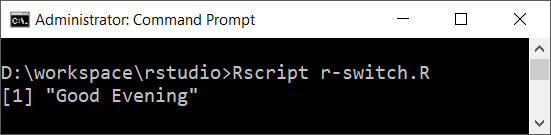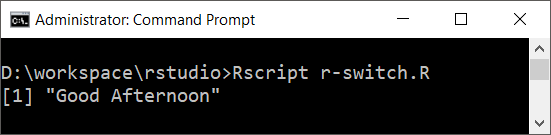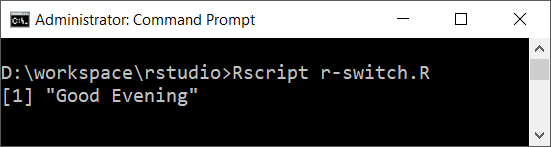In this tutorial, we shall learn R switch statement based on index and matching value, its syntax and examples to demonstrate the realization of decision making using switch statement.

## R switch statement

R switch statement provides decision making capability. It allows to select one of the cases based on the value of an expression.

There are two ways in which one of the cases is selected.

1. Based on Index – If the cases are just values (like a Character Vector), and if the expression is evaluated to a number, the expression’s result is used as index to select the case.
2. Based on Matching Value – If the cases have both case value and output value like [“case_1″=”value1”], then the expression value is matched against case values. When there is a match with the case, the corresponding value is the output.

We shall see about these two ways in detail.

## R switch statement – Based on Index

Syntax

 switch(expression, list)

### Example 1

Output### Example 2

In the following R switch statement, we used expression with multiple variables.

Output## R switch statement – Based on Matching Value

Syntax

 switch(expression, case1=value1, case2=value2, …, caseN=valueN)

### Example 3

Output### Example 4

In the following R switch statement, we used a string concatenation expression.

Output### Conclusion

In this R Tutorial, we have learnt R switch statement, its syntax and examples to demonstrate the realisation of decision making using switch statement.